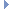OpenMM
HarmonicBondForce Class Reference

This class implements an interaction between pairs of particles that varies harmonically with the distance between them. More...Inheritance diagram for HarmonicBondForce:

List of all members.

## Public Member Functions

def getNumBonds
getNumBonds(self) -> int
addBond(self, particle1, particle2, length, k) -> int
def getBondParameters
Get the force field parameters for a bond term.
def setBondParameters
Set the force field parameters for a bond term.
def updateParametersInContext
Update the per-bond parameters in a Context to match those stored in this Force object.
def usesPeriodicBoundaryConditions
usesPeriodicBoundaryConditions(self) -> bool
def __init__
__init__(self) -> HarmonicBondForce __init__(self, other) -> HarmonicBondForce

this

## Detailed Description

This class implements an interaction between pairs of particles that varies harmonically with the distance between them.

To use it, create a HarmonicBondForce object then call addBond() once for each bond. After a bond has been added, you can modify its force field parameters by calling setBondParameters(). This will have no effect on Contexts that already exist unless you call updateParametersInContext().

## Constructor & Destructor Documentation

 def __init__ ( self, args )

__init__(self) -> HarmonicBondForce __init__(self, other) -> HarmonicBondForce

Create a HarmonicBondForce.

## Member Function Documentation

 def addBond ( self, particle1, particle2, length, k )

addBond(self, particle1, particle2, length, k) -> int

Add a bond term to the force field.

Parameters:
 particle1 (int) the index of the first particle connected by the bond particle2 (int) the index of the second particle connected by the bond length (double) the equilibrium length of the bond, measured in nm k (double) the harmonic force constant for the bond, measured in kJ/mol/nm^2
Returns:
(int) the index of the bond that was added
 def getBondParameters ( self, index )

Get the force field parameters for a bond term.

Parameters:
 index (int) the index of the bond for which to get parameters
Returns:
(int) the index of the first particle connected by the bond
(int) the index of the second particle connected by the bond
(double) the equilibrium length of the bond, measured in nm
(double) the harmonic force constant for the bond, measured in kJ/mol/nm^2
 def getNumBonds ( self )

getNumBonds(self) -> int

Get the number of harmonic bond stretch terms in the potential function

 def setBondParameters ( self, index, particle1, particle2, length, k )

Set the force field parameters for a bond term.

Parameters:
 index (int) the index of the bond for which to set parameters particle1 (int) the index of the first particle connected by the bond particle2 (int) the index of the second particle connected by the bond length (double) the equilibrium length of the bond, measured in nm k (double) the harmonic force constant for the bond, measured in kJ/mol/nm^2
 def updateParametersInContext ( self, context )

Update the per-bond parameters in a Context to match those stored in this Force object.

This method provides an efficient method to update certain parameters in an existing Context without needing to reinitialize it. Simply call setBondParameters() to modify this object's parameters, then call updateParametersInContext() to copy them over to the Context.

The only information this method updates is the values of per-bond parameters. The set of particles involved in a bond cannot be changed, nor can new bonds be added.

 def usesPeriodicBoundaryConditions ( self )

usesPeriodicBoundaryConditions(self) -> bool

Returns whether or not this force makes use of periodic boundary conditions.

Returns:
(bool) true if force uses PBC and false otherwise

Reimplemented from Force.

## Member Data Documentation

Reimplemented from Force.

The documentation for this class was generated from the following file:
• openmm.py